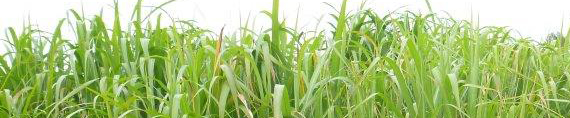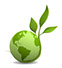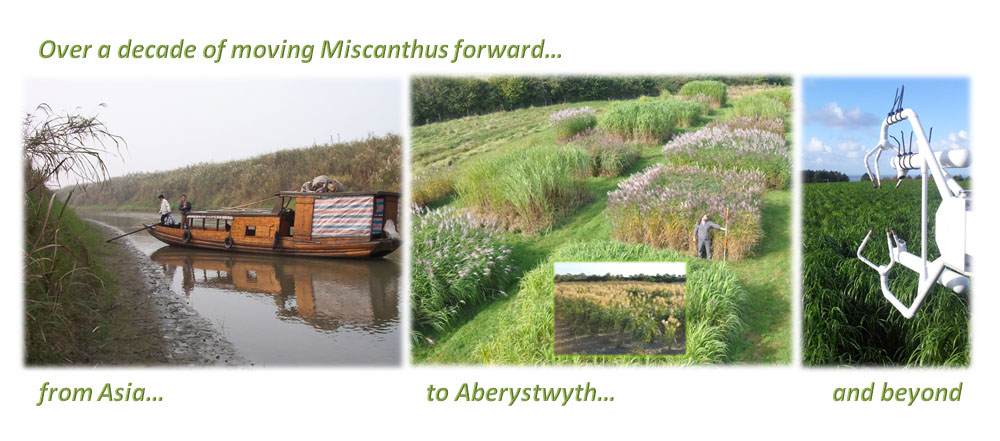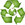M  i  s  c  a  n  t  h  u  s    B  r  e  e  d  i  n  gM i s c a n t h u s   B r e e d i n g   t o   p r o v i d e   s u s t a i n a b l e   g l o b a l   b i o e n e r g y   f o r   t h e   f u t u r e